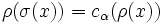Conjugacy-separable with only finitely many prime divisors of orders of elements implies every extensible automorphism is class-preserving

Statement

Suppose$G$ is a Conjugacy-separable group (?): in other words, given any two elements$x,y$ of$G$ that are not conjugate, there exists a normal subgroup of finite index$N$ in$G$ such that the images of$x,y$ in$G/N$ are not conjugate in$G/N$.

Suppose, further, that the set of primes$p$ that divide the order of some non-identity element of$G$ is finite.

Then, if$\sigma$ is an Extensible automorphism (?) of$G$,$\sigma$ is a Class-preserving automorphism (?) of$G$.

Proof

Given: A conjugacy-separable group$G$ with only finitely many primes$p$ dividing the orders of elements of$G$. An extensible automorphism$\sigma$ of$G$. Two elements$x,y$ of$G$ that are not conjugate.

To prove:$\sigma$ cannot send$x$ to$y$.

Proof:

1. There exists a normal subgroup$N$ of finite index in$G$ such that the images of$x$ and$y$ are not conjugate in$N$: This follows from the definition of conjugacy-separable.
2. Let$\overline{G} = G/N$. Then, there exists a prime$p$ such that the field of$p$ elements is sufficiently large for$\overline{G}$, and such that$p$ does not divide the order of any element of$G$: By fact (2), there are infinitely many sufficiently large prime fields for$\overline{G}$, i.e., there are infinitely many primes$p$ for which the corresponding prime field is sufficiently large for$G$. Since there are only finitely many prime divisors of orders of elements, we can find a prime$p$ not among any of these divisors such that the corresponding prime field is sufficiently large.
3. The field of$p$ elements is a class-separating field for$\overline{G}$. In particular, there is a finite-dimensional linear representation$\rho_1:\overline{G} \to GL(V)$ of$\overline{G}$ over this field such that$\rho_1(\overline{x})$ and$\rho_1(\overline{y})$ are not conjugate: This follows from fact (3).
4.$\sigma$ is linearly pushforwardable over the prime field with$p$ elements, for the$p$ chosen above. In particular, if$\sigma(x) = y$, then$\rho(x)$ and$\rho(y)$ are conjugate for any representation$\rho$ over this field: Let$\rho$ be a representation of$G$ over this field. Let$V$ be the corresponding vector space and$H = V \rtimes G$ the semidirect product for the action. Since$p$ does not divide the order of any element of$G$,$V$ is the set of elements of$H$ of order dividing$p$. In particular,$V$ is characteristic in$H$, and thus, if$\sigma$ extends to an automorphism$\sigma'$ of$H$, then$\sigma'$ also restricts to an automorphism$\alpha$ of$V$. Fact (1) thus yields that$\rho \circ \sigma = c_\alpha \circ \rho$, so$\sigma$ is linearly pushforwardable over the field of$p$ elements. In particular, if$\sigma(x) = y$, then$\rho(\sigma(x)) = c_\alpha(\rho(x))$, so$\rho(y)$ is conjugate to$\rho(x)$ by$\alpha$.
5. Let$\rho_1$ be the linear representation chosen in step (3), and let$\rho = \rho_1 \circ p$ where$p:G \to G/N = \overline{G}$ is the quotient map. Then,$\rho(x)$ and$\rho(y)$ are not conjugate in the general linear group$GL(V)$. However, by step (4), we have that$\sigma$ is linearly pushforwardable, so if$\sigma(x) = y$, then$\rho(x)$ and$\rho(y)$ are conjugate. This gives a contradiction, so we cannot have$\sigma(x) = y$, and we are done.